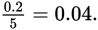# SAT Math Multiple Choice Question 366: Answer and Explanation

### Test Information

Question: 366

6. If 0.002 ≤ x ≤ 0.2 and 5 ≤ y ≤ 25, what is the maximum value of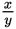?

• A. 0.04
• B. 0.4
• C. 4
• D. 40

Strategic Advice: The question is asking about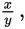so think about how fractions work. Larger numerators result in larger values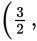for example, is greater thanand smaller denominators result in larger values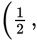for example, is greater than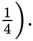Getting to the Answer: The largest possible value ofis found by choosing the largest possible value for x and the smallest possible value for y: